Hello. How are you all? It's always a pleasure for me to write on the blog. The community is growing, although it's not very active yet (please comment, write to me, let's learn together). Today, we continue our discussion on inferential statistics. In the article "Inferential Statistics - Part1", we introduced inferential statistics with key concepts like "estimate" and "estimator". We explained how inferential statistics allow us to estimate a parameter of a population based on data from one or more samples taken from it, thanks to the "Central Limit Theorem". In this second part, and heads up, there will be a third part and maybe even a fourth, we will talk about the very important "Hypothesis Test".

Despite our confidence in the Central Limit Theorem, estimating a population parameter is, in itself, a hypothesis. When faced with a hypothesis, it's natural to ask:

Can we trust this hypothesis?

To answer this question, it's possible to use a statistical test known as the Hypothesis Test. A hypothesis test is an investigative strategy that helps us make decisions. Specifically, it lets us decide whether to reject or not reject a certain research hypothesis.

There are various types of hypothesis tests, and each one has certain assumptions that need to be met to use them. Generally, a hypothesis test examines a specific hypothesis called the "Null Hypothesis," denoted as H0. The null hypothesis is assumed to be true until proven otherwise. By testing it, we try to determine if what it claims or the estimate it provides is accurate or it's wrong, perhaps due to random chance. If we find out that what H0 claims is due to chance or it's simply wrong, we can't accept it as true. Therefore, we decide to reject it and accept the "Alternative Hypothesis," denoted as H1. This hypothesis claims the opposite of H0. So, if H0 claims that it's raining today, H1 would claim that it's NOT raining today. Remember that H0 is the actual hypothesis under investigation, so the study hypothesis.

The hypothesis system made up of H0 and H1 is more elegantly displayed in the image below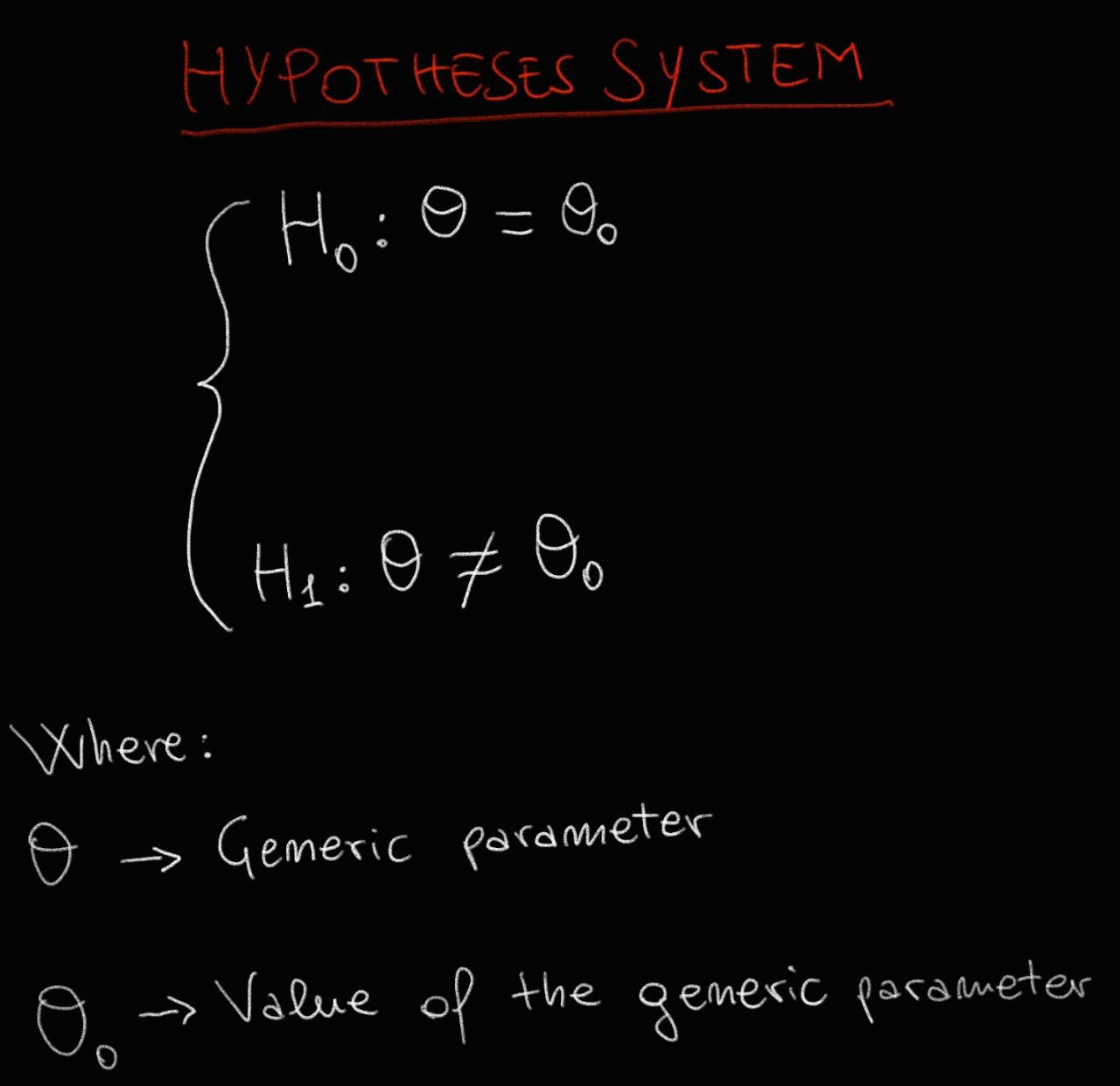The ingredients needed to conduct a hypothesis test are:

• A set of hypotheses (H0 and H1);
• A test statistic, which is a quantity calculated using a formula;
• A decision rule. Based on the test statistic's value compared to a predetermined threshold value, we decide whether to reject H0 or not;
• An accepted error level. This is a numerical value showing the degree of error we're willing to accept when rejecting H0. This error level, also known as significance, is represented by the letter alpha. Using an alpha value of 0.05 means we accept a 5% chance of making an error when rejecting H0. Specifically, there are two types of errors in this context: Type I Error and Type II Error. The first is rejecting H0 when it should be accepted, and the second is NOT rejecting H0 when it should be. To clarify, alpha represents the accepted probability of making a Type I error, while the letter Beta represents the accepted probability of making a Type II error;• A value that quantifies the probability, upon repeating the test multiple times, of obtaining a result that's the same as or more rare than the observed one;
• A confidence interval, which is a numerical range with a lower and upper limit, within which we believe, with a certain level of confidence (probability), the true value of the estimated parameter lies. This "ingredient" is actually optional;

The workflow of a hypothesis test is illustrated in the image below: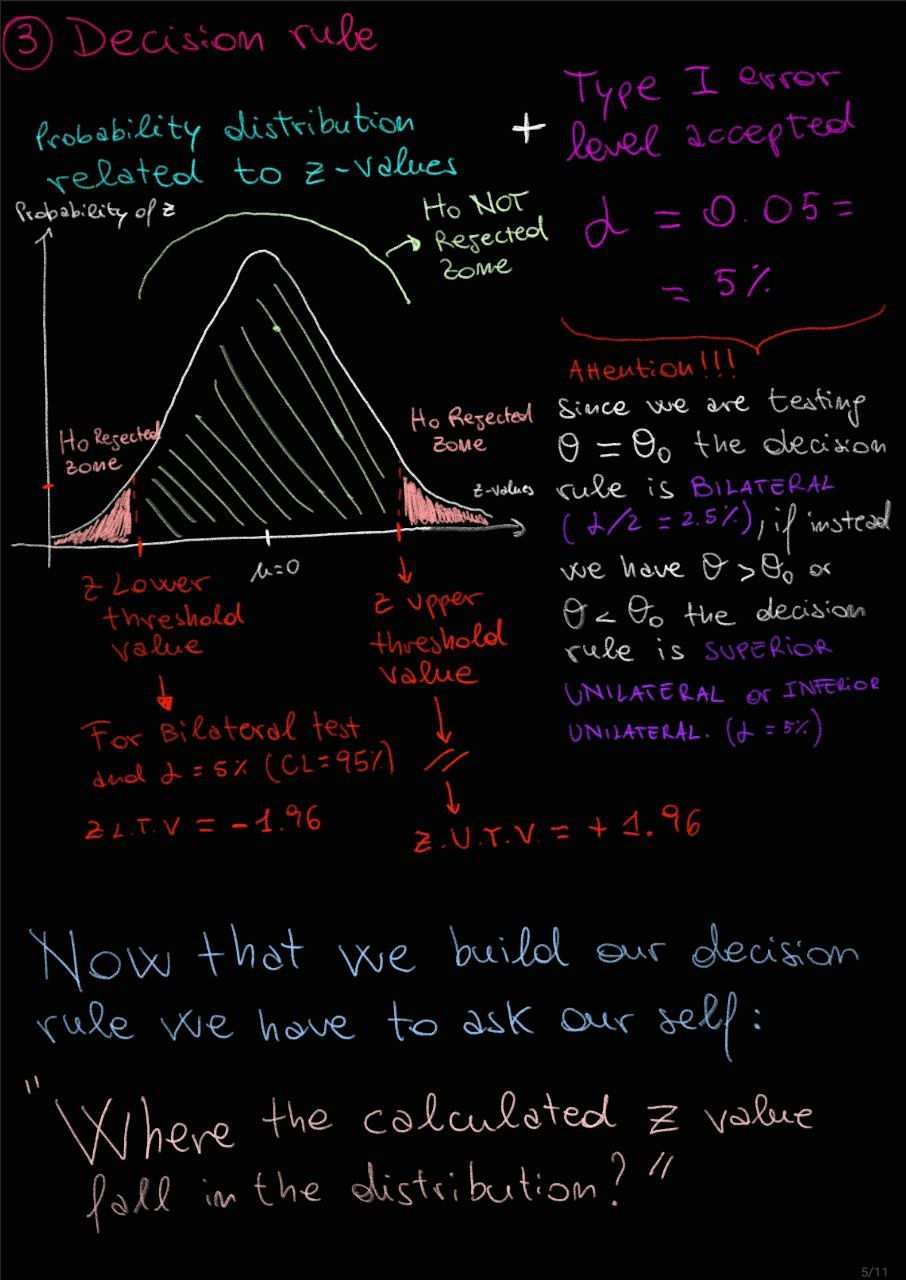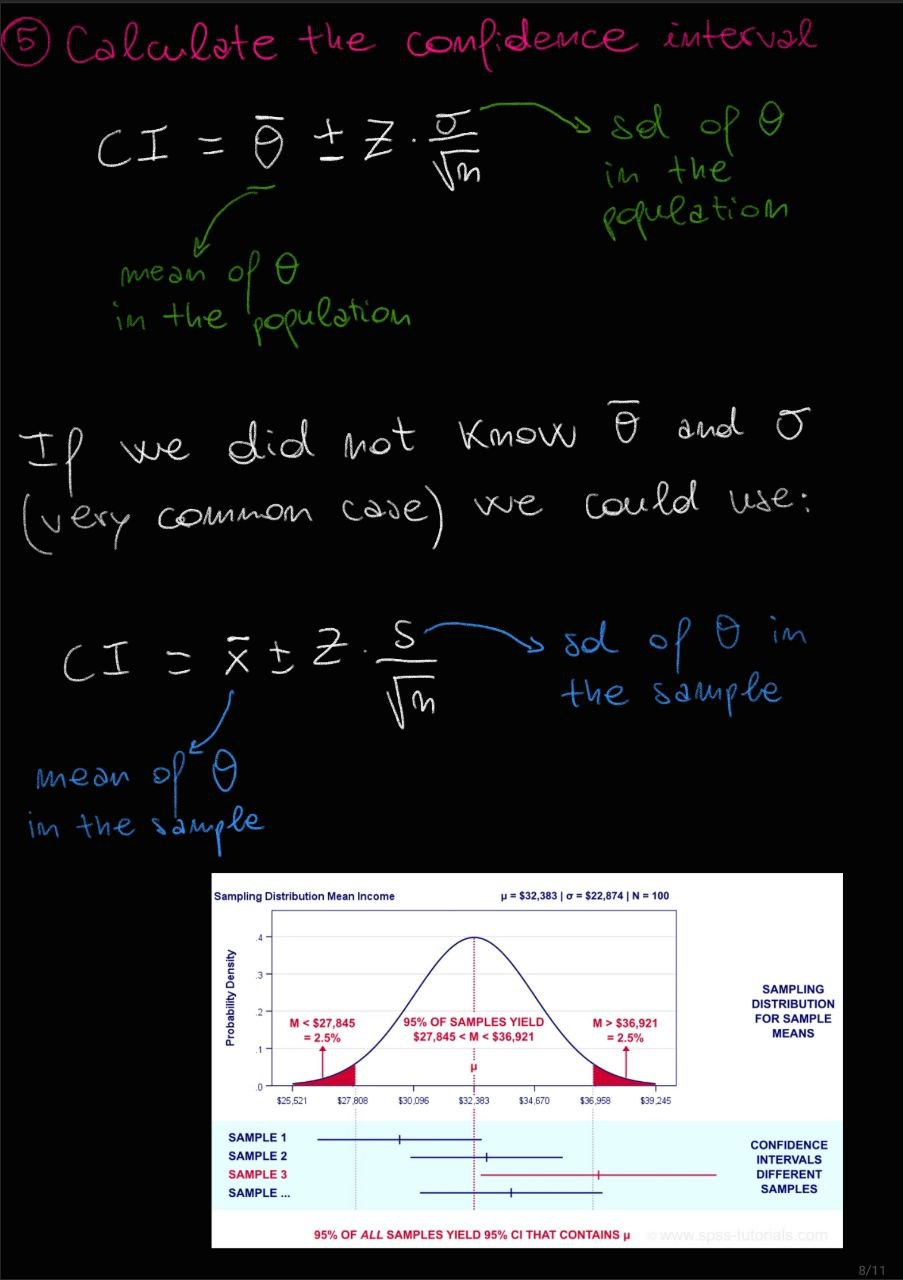### Important Clarifications

• If, in the hypothesis system created, the relationship between the general parameter and the hypothesized parameter value is of the "different from" type, we are dealing with a two-tailed test. In this case, the significance level alpha is divided between the two tails of the standard normal distribution of probability related to the z values. If we have a "greater than" relationship, we are looking at a right-tailed hypothesis test, where the significance level alpha is entirely placed on the right tail. Conversely, if the relationship is of the "less than" type, we have a left-tailed hypothesis test with alpha entirely placed on the left tail of the aforementioned distribution.• When constructing the decision rule, remember that alpha corresponds to the accepted probability of committing a Type I error (also defined as the test's significance level). Beta corresponds to the accepted probability of committing a Type II error. Meanwhile, 1-alpha represents the confidence level, which is the probability of making a correct decision in our hypothesis test. It's crucial not to confuse the confidence interval with the confidence level. The former indicates a range of z-values with set lower and upper bounds, while the latter expresses a probability. More precisely, the "confidence level" is the likelihood that a confidence interval, containing a population's parameters or a statistical test result, genuinely captures the real parameter values or desired result when the sampling process is repeated many times. In other words, the confidence level measures how "sure" or "confident" you can be that the confidence interval or the outcome of a statistical test includes the actual population parameter value or reflects the true situation, based on repeated random samples. A typical confidence level is 95%, suggesting that if the sampling process were repeated many times, we'd expect 95% of the confidence intervals or test results to truly encompass the actual value or reflect the real situation. However, it's essential to note that a 95% confidence level also means there's a 5% chance of obtaining a confidence interval or test result that doesn't capture the real parameter value or could be affected by sampling error. Hence, choosing the confidence level should consider the desired accuracy's trade-off with the error probability.• The critical threshold value, z, that defines the entry into the H0 rejection zone can be determined using a standard normal z distribution table, or you can use statistical software. The general formula to calculate the critical value is:
For a one-tailed test (on the right):
z_critical = +Zα (where "" signifies the intent to find the z value corresponding to α)

For a one-tailed test (on the left):
z_critical = -Z_α (for the left z value, add a minus sign)

For a two-tailed test (both left and right):
zcritical = +-Z(α/2)

To better understand how to identify the critical z values, I suggest watching the following video or read this.

However, there are some frequently used z-cratic values​​listed in the table below: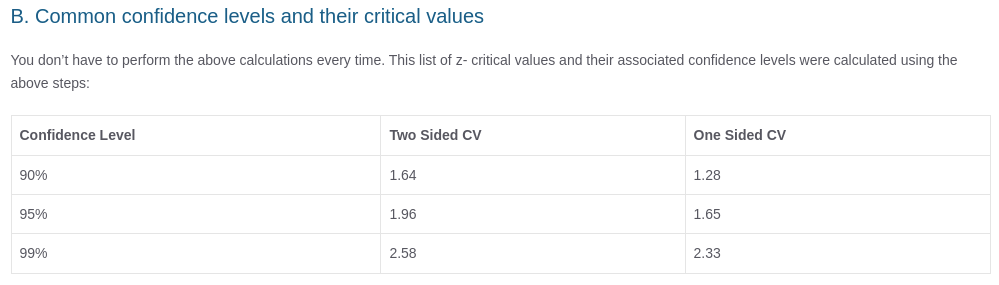• The p-value is a highly debated and intriguing concept. The p-value in a hypothesis test is a measure of the evidence against a null hypothesis. We could define the p-value as the probability of observing a test statistic as extreme as, or more extreme than, the statistic computed from the sample, given that the null hypothesis is true. The p-value must be compared with alpha value (α) indeed:
• If p-value ≤ α : Reject the null hypothesis (H0).
• If p-value > α : Fail to reject the null hypothesis (H0).It's crucial to understand that "failing to reject" doesn't equate to "accepting." It merely indicates a lack of sufficient statistical evidence to reject H0, rather than a confirmation of H0's truth. Additionally, p-values don't offer the probability of either hypothesis being true; they merely provide evidence against the null. When conducting multiple hypothesis tests, it's essential to be aware of the risk of Type I errors (false positives). Techniques such as the Bonferroni or FDR correction can help mitigate this risk.

For a clearer understanding of this concept, I recommend watching this video:

#### Bonus Tips:

As a bioinformatician, I frequently work with p-values. In RNAseq analysis, for instance, the p-value helps determine the statistical significance of the log2FC value, which measures the difference in gene expression across multiple conditions.
In the context of RNA-seq analysis, differential gene expression typically involves comparing gene expression levels between two or more conditions (e.g., treated vs. untreated samples) to identify genes that are differentially expressed (DE) between the conditions.

Here is a brief summary of how the p value comes into play in RNAseq analysis:

1. Differential Expression: The main aim is to determine which genes have significantly different expression levels between conditions.

2. Log2 Fold Change: This is a measure of the magnitude of differential expression. A log2 fold change value of 1 means the gene is 2-fold upregulated in one condition compared to another, while a value of -1 means it's 2-fold downregulated.

3. p-value: This indicates the statistical significance of the observed differential expression. A smaller p-value indicates that the observed difference in gene expression is less likely to have occurred by random chance.

4. Filtering DE genes with a p-value < 0.05: This means you're selecting genes that have a statistically significant differential expression with a p-value less than 0.05 (α). This is a common threshold, but in many RNA-seq analyses, additional adjustments for multiple testing (like FDR) are also applied due to the large number of genes being tested.

5. Relation to Log2 Fold Change: While the p-value tells you if a gene's differential expression is statistically significant, the log2 fold change tells you about the magnitude of that change. Sometimes, researchers might filter genes not only based on p-value but also by setting a threshold on the fold change (e.g., genes with |log2FC| > 1, which corresponds to 2-fold up or downregulation).

In summary, in RNA-seq analysis, genes that are filtered with a p-value (related to differential expression) less than 0.05 are considered to be statistically significantly differentially expressed. However, it's also important to consider the magnitude (fold change) and direction (up or downregulated) of the expression change, as well as adjust for multiple testing to get a comprehensive view of the differential expression landscape.

### Pay Attention to Assumptions

As previously mentioned, there are various types of test statistic, and each comes with certain assumptions that must be met to ensure their proper use in hypothesis testing. In the workflow depicted in the image above, we used the Z-test. But what are the conditions that must be met to use this type of test statistic in hypothesis testing?

The main assumptions for using a Z-test are:

1. Random Sampling: The sample data should be obtained using a method that ensures that every individual observation has an equal chance of being included. This often means using simple random sampling.

2. Normal Distribution or Large Sample Size:

• If you're testing means, the distribution of the population from which the sample is drawn should be approximately normal. This is especially important if the sample size is small.
• If the population distribution is not known or is not normal, the Central Limit Theorem (CLT) tells us that for sufficiently large sample sizes (usually a sample size of 30 or more is considered large enough), the sample means will be approximately normally distributed even if the population is not.
3. Known Population Standard Deviation: For a true z-test, you should know the standard deviation of the population (σ). If you don’t, and you instead use the sample standard deviation (s), you're technically conducting a t-test, not a z-test.

4. Independence: Each sampled observation should be independent of any other observation. This means that the occurrence of one event doesn't affect the occurrence of another. In most practical scenarios, if you're sampling without replacement, a guideline is that if your sample size is less than 10% of the population, you can assume independence.

5. Scale of Measurement: The scale of measurement for the data should be at least interval (or ratio). This means that the differences between data points are consistent and meaningful. Examples include age, height, weight, and scores on a test.

6. Simple Hypothesis Testing: You are comparing observed data to a single value or comparing the data between two groups. If you have multiple groups or categories, you might need to use other statistical tests like ANOVA.

Make sure to always check the assumptions before you conduct the test to ensure the results are valid and meaningful.

Once you've verified that all these assumptions are met, you can proceed with executing the Z-test to test your hypotheses. But what happens if one of these conditions isn't met? For instance, how should we handle data that isn't normally distributed?

Don't despair, as we can use another type of test better suited to the conditions of our study.
In fact, when dealing with a sample size that's too small (< 30 samples) and/or the population variance is unknown and/or the data doesn't follow a normal distribution of probability values, the T-test can be used instead of the Z-test.

The t-value is used during the decision phase of the hypothesis test, much like the z-value, but they are distinct. It's essential to clarify that the probability values corresponding to t-values follow what's known as the t-distribution. This distribution is similar to a standard normal distribution (like the one for z-values). It's centered and symmetrical around zero (mean = 0), but its variance depends on its degrees of freedom. As degrees of freedom increases, the t-distribution converges towards a standard normal distribution.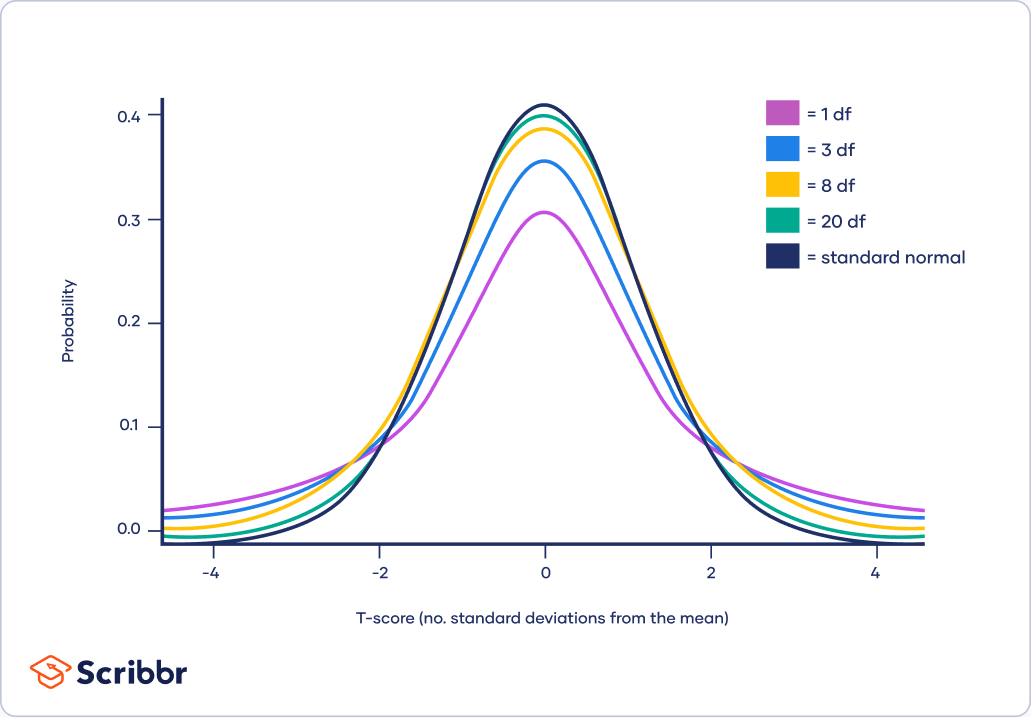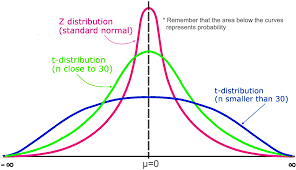The formula to calculate the t value is shown below:Now let's get our hands dirty with some code by doing some hypothesis testing examples.

##### Hypothesis test using z statistic test:
``````### Context ----

# In literature is known that IQ in the human population has a normal probability distribution with a mean of 100 and a standard deviation of 15.
# A teacher suspects that class A have an average intelligence, so let's go and test this hypothesis.

### Data ----

# Set the seed for reproducibility
set.seed(23)

# Population QI probability distribution visualization
QI <- rnorm(100000,
mean = 100,
sd = 15)
plot(density(QI))
abline(v = mean(QI), col = "red") # Add a mean line to the plot
````````````# Let's create class A dataframe with QI value for each student of class A.
# Number of rows
n <- 35 # sample size

# Generate random QI values between 80 and 110
# These will be the QI value of each student
qi_values <- runif(n, min = 80, max = 110)

# Create a dataframe
df_class_A <- data.frame(QI_value = qi_values)

# Print the dataframe
print(df_class_A)

### Define system of hypotheses ----

###############################
#  H0: class_A_mean_QI = 100  #
#                             #
#  H1: class_A_mean_QI != 100 #
###############################

# Important !!!!!!

# Due to relation in terms of class_A_mean_QI = 100, we are in front of a two tailed hypothesis test.

# The esteem of class A QI mean is: 94.43
esteem <- round(mean(df_class_A\$QI_value), 2)
print(esteem)

### Calculate the statistic test using z-test ----

# Remember the formula of z-test? In our case became:

# z = (esteem_class_A_mean_QI - population_mean_QI)/population_sd_QI/sqrt(n)

z_test <- function(esteem, hypothesized_value, pop_sd, n){
z <- (esteem - hypothesized_value)/(pop_sd/sqrt(n))
}

# Use the function with our data
z_value <- z_test(esteem = esteem, hypothesized_value = 100, pop_sd = 15, n = length(df_class_A\$QI_value))
print(z_value)

### Define the decision rule ----

# Define the significant level alpha
alpha <- 0.05

# Define the z critical values
critical_z_value <- qnorm(c(alpha, 1-alpha))
print(critical_z_value)

# Visualize the z probability distribution
z_prob_distribution <- rnorm(10000000, 0, 1)
plot_z <- plot(density(z_prob_distribution))
abline(v=critical_z_value, col='red')

# Let's see where the calculated z value fall into the distribution with respect to the critical z values
points(x=z_value, y=0, pch=20, col=4, cex=3)
``````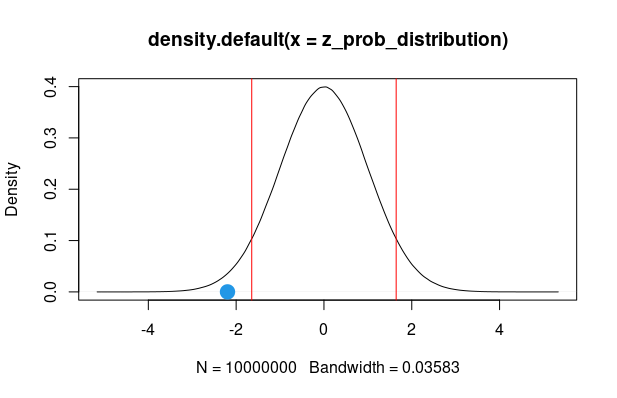``````### So what?

# From the graph of the probability distribution of the z values, we note that the calculated z value falls
# in the region of rejection of H0, therefore we can say that the teacher is wrong.
# Class A students do not have average intelligence.

# But the teacher is still not convinced, she thinks that the results of the z-test may be wrong or due to chance.
# So let's use the pvalue to see whether the data provides enough evidence to support a rejection of the null hypothesis in favor of an alternative hypothesis.

### Calculate p-value ----

# To calculate the p-value associated with a z-score in R, we can use the pnorm() function
# Calculate p-value for two-tailed test
p_value <- 2 * (1 - pnorm(abs(z_value)))
print(p_value)

# Related p_value and alpha value
if (p_value < alpha){
print("The comparison between pvalue and aplha value suggests that we should reject H0.")
} else{
print("The comparison between pvalue and aplha value suggests that we should NOT reject H0.")
}

### Another help for the decision to make in hypothesis testing comes from the confidence interval.

# Remember!!!

# Confidence intervals provide a range of values within which a population parameter,
# such as a population mean or proportion, is likely to fall.
# Confidence intervals provide a range of plausible values for a population parameter, such as a population mean.
# For example. If the null hypothesis is that a population parameter equals a specific value, you can use a confidence interval
# to see if that specific value falls within the interval. If it does, it suggests that the null hypothesis is plausible.
# If it doesn't, it suggests that the null hypothesis is unlikely, which may lead to rejecting it in favor of the alternative hypothesis.
# In summary, confidence intervals provide information about the precision and range of plausible values for a population parameter,
# which can be used in conjunction with hypothesis testing to make decisions about whether to reject or not reject the null hypothesis
# based on the evidence from sample data.

sd = 15
Upper_limit <- esteem + (critical_z_value * sd/sqrt(n))
Lower_limit <- esteem - (critical_z_value * sd/sqrt(n))
CI <- c(Lower_limit, Upper_limit)
plot(density(QI))
abline(v = mean(QI), col = "black") # Add a mean line to the plot
abline(v = CI, col = "violet") # Add a CI lines to the plot
``````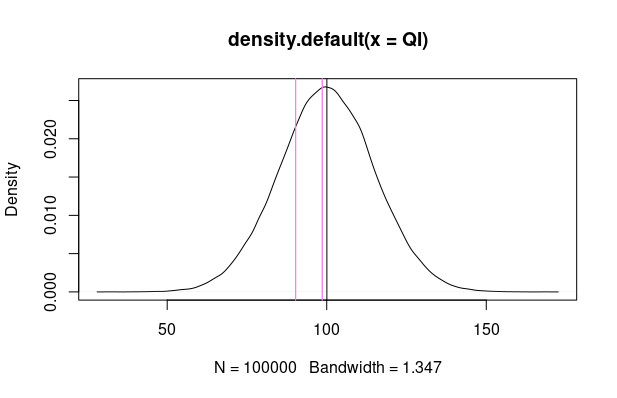``````################################################################################################################################################################
# ---- CONCLUSIONS ----
# In conclusion we observe that:
# 1) The test statistic (z-value calculated) falls within the rejection zone of H0. This tells us to reject H0.
# 2) The p value is smaller than alpha and this suggests that we must reject H0.
# 3) The calculated confidence interval does not contain the value of the parameter under the null hypothesis. This tells us we must reject H0.
# Therefore we can say that the teacher is wrong. Class A students do not have average intelligence.
################################################################################################################################################################

# A code bonus ----
# In R you can speed up hypothesis testing using the package TeachingDemos.
# Performe a z-test with TeachingDemos.
# pay attention to the 'alternatives' parameter of the function. This allows us to specify whether we are considering a One-tailed or Two-tailed hypothesis test.
# For example, if we wanted to test the hypothesis that class A has an average intelligence greater than the average intelligence of the population we could use the
# function TeachingDemos::z.test in the following way:

TeachingDemos::z.test(df_class_A\$QI_value, mu = 100, stdev = 15, n = 35, alternative = "greater", conf.level = 0.95)

### ------------------- ###
# Some clarifications:

# - If the standard deviation of the population is unknown, we should use t-test instead of z-test.
# - If the sample size is smaller the 30, we should use t-test instead of z-test.
# - If the probability distribution related to the variable values is NOT normal, we should use t-test instead of z-test.
``````
##### Hypothesis test using t statistic test:
``````### Context ----

# We're flipping a coin to decide who gets to clean the house today.
# If heads you clean it, if tails I clean it.
# However, one of you hypothesizes that the coin used is fake. Let's test this hypothesis.

### Data ----

# Set the seed for reproducibility
set.seed(26)

# Flipping the coin 10 times we get the following dataframe:
library('tidyverse')

df <- table(Coin_flip)/length(Coin_flip)
df <- as.data.frame(df)
n <- length(Coin_flip)

# Look at the probability distribution of sample coin flip variable
plot(density(df\$Freq))
abline(v = mean(df\$Freq), col = "red")

# The probability distribution of sample coin flip variable is a Binomial Distribution.
# Since it is not a normal distribution and also has a sample size smaller than 30,
# we cannot use the z test statistic.
``````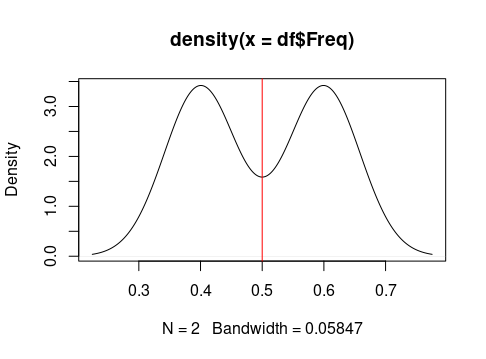``````### Define system of hypotheses ----

# To be fair a coin flip must show a proportion of 0.5 getting Heads and 0.5 getting Tails.
# Then considering arbitrarily testing the proportion of Heads or Tails obtained from the coin toss,
# the hypotheses system should be:

#############################################################
#  H0: Proportion of Head = 0.5 (fair coin proportion)
#  H1: Proportion of Head != 0.5 (NOT fair coin proportion) #############################################################

# IMPORTANT !!!!!

# We need to convert the 'Heads' and 'Tails' data into numbers as we cannot do mathematical operations on the characters.
print(Coin_flip)
print(numeric_vector_data)

# Let's define the values ​​to feed the t test statistic.

# Let's calculate the t-test statistic:
print(T_stat)

# Calculate critical (or threshold) t value related to a two tailed hypotesis test
# and considering alpha = 0.05 which will be distributed on the two tails with values ​​of 0.025
# and considering n-1 degrees of freedom.
degrees_of_freedom <- n-1
alpha <- 0.05
critical_t_value <- qt((alpha/2), degrees_of_freedom)
print(critical_t_value)
t_value_thresholds <- c(critical_t_value, abs(critical_t_value))
print(t_value_thresholds)

if (T_stat > t_value_thresholds | T_stat < t_value_thresholds){
print('We should NOT reject H0')
} else{
print('We should reject H0')
}

# Let's see where the calculated t value falls in a t-student probability distribution with n-1 degrees of freedom, with respect to the threshold t values.
plot(density(rt(100000, degrees_of_freedom)), xlim=c(-5,5))
abline(v = t_value_thresholds, col = 'red')
points(x=T_stat, y=0, pch=20, col=4, cex=3)
````````````# Let's create confidence intervals
CI <- c(Lower_limit, Upper_limit)

# Calculate p-value for two-tailed test
p_value <- 2 * (1 - pnorm(abs(T_stat)))
print(p_value)

# Related p_value and alpha value
if (p_value < alpha){
print("The comparison between pvalue and aplha value suggests that we should reject H0.")
} else{
print("The comparison between pvalue and aplha value suggests that we should NOT reject H0.")
}

#########################################################################################################################################################################
# ---- CONCLUSIONS ----
# In conclusion we observe that:
# 1) The test statistic (T_stat calculated) falls within the NOT rejection zone of H0 (less precisely called the acceptance zone of H0. This tells us to NOT reject H0.
# 2) The p value is greater than alpha and this suggests that we must NOT reject H0.
# 3) The calculated confidence interval contain the value of the parameter under the null hypothesis. This tells us we must NOT reject H0.
# Therefore we can say that the coin is fair. Proportion of Head is almost equal to 0.5.
#########################################################################################################################################################################

### Bonus code ---
# Using t.test() R base function
TeachingDemos::t.test(numeric_vector_data,